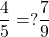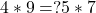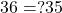## Neha used 4 bananas and 5 oranges in her fruit salad. Daniel used 7 bananas and 9 oranges. Did Neha and Daniel use the same ratio of bananas

Question

Neha used 4 bananas and 5 oranges in her fruit salad. Daniel used 7 bananas and 9 oranges. Did Neha and Daniel use the same ratio of bananas to oranges? If not, who used the greater ratio of bananas to oranges? Use cross multiplication to see if the ratios are the same. Help me pleasee!! This assignment is due tmr!!! :C I will name u Brainliest if you answer this correctly!

in progress 0
3 months 2021-08-02T15:16:26+00:00 1 Answers 6 views 0

Neha and Daniel do not use the same ratio of bananas to oranges

Neha used the grater ratio of bananas to oranges

Step-by-step explanation:

Ratios

The ratio is expressed as a:b, where a and b are whole numbers.

Neha used 4 bananas and 5 oranges in her fruit salad. Daniel used 7 bananas and 9 oranges in his.

We use cross multiplication to find if the ratios of bananas to oranges are the same.

Neha s ratio is 4:5

Daniel s ratio is 7:9

To compare both ratios we use the expression:The question mark means equality could be true or false.

The cross multiplication consists of multiplying the denominator of one side by the numerator of the other side:Operating:The equality is false, thus

Neha and Daniel do not use the same ratio of bananas to oranges

Since the left side of the equality is greater than the second:

Neha used the grater ratio of bananas to oranges